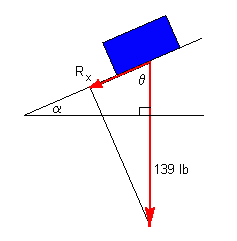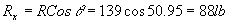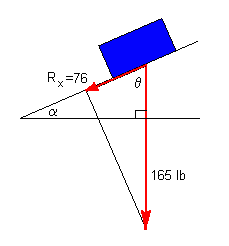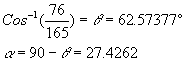# Occupational Health and Safety: Ramp Design

At a local seed and fertilizer company, fertilizer is funneled into cart-like containers which must be pushed by an employee up an inclined ramp to the next processing station. Suppose regulations state that the maximum force an employee can exert in a repetitive task is 80. pounds (note: 2 significant figures).

1. If the plane is inclined at an angle of 39° 3′ to the horizontal, determine whether the force required to push a filled cart weighing 139 pounds up the ramp exceeds the regulation. (Assume the force of friction is negligible.)
2. The company wishes to redesign the ramp so that the following conditions are met:
• the maximum weight in the cart is 165 pounds
• the maximum push required by an employee is at least 5% below the regulation.

At what angle must the new ramp be inclined to the horizontal?

## Solution

1. First convert α= 39° 3′ to decimal degrees = (by calculator)=39.05°. Also θ= 90 - 39.05 = 50.95°

Recall that the x component of a vector is R cos(θ) and the y component of a vector is R sin(θ).ThenThe force required to push the cart is 88lb which exceeds the regulation.

2. 95% of 80 lb is 76 lb which is 5% below the regulation of 80lb.Thus the ramp should be inclined at an angle of 27° with the horizontal.Written by Louise Routledge, September 1, 1998